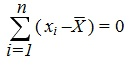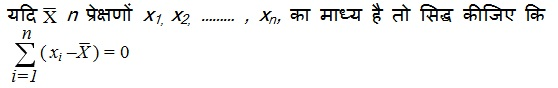Study Materials: ncert solutions

Our ncert solutions for Exercise 14.4 Class 9 maths 14. Statistics - ncert solutions - Toppers Study is the best material for English Medium students cbse board and other state boards students.

Solutions ⇒ Class 9th ⇒ Mathematics ⇒ 14. Statistics

# Exercise 14.4 Class 9 maths 14. Statistics - ncert solutions - Toppers Study

Topper Study classes prepares ncert solutions on practical base problems and comes out with the best result that helps the students and teachers as well as tutors and so many ecademic coaching classes that they need in practical life. Our ncert solutions for Exercise 14.4 Class 9 maths 14. Statistics - ncert solutions - Toppers Study is the best material for English Medium students cbse board and other state boards students.

## Exercise 14.4 Class 9 maths 14. Statistics - ncert solutions - Toppers Study

CBSE board students who preparing for class 9 ncert solutions maths and Mathematics solved exercise chapter 14. Statistics available and this helps in upcoming exams 2023-2024.

### You can Find Mathematics solution Class 9 Chapter 14. Statistics

• All Chapter review quick revision notes for chapter 14. Statistics Class 9
• NCERT Solutions And Textual questions Answers Class 9 Mathematics
• Extra NCERT Book questions Answers Class 9 Mathematics
• Importatnt key points with additional Assignment and questions bank solved.

NCERT Solutions do not only help you to cover your syllabus but also will give to textual support in exams 2023-2024 to complete exercise 14.4 maths class 9 chapter 14 in english medium. So revise and practice these all cbse study materials like class 9 maths chapter 14.4 in english ncert book. Also ensure to repractice all syllabus within time or before board exams for ncert class 9 maths ex 14.4 in english.

See all solutions for class 9 maths chapter 14 exercise 14 in english medium solved questions with answers.

### Exercise 14.4 class 9 Mathematics Chapter 14. Statistics

• Exercise 14.4 Class 9 Maths 14. Statistics - Ncert Solutions - Toppers Study
• Class 9 Ncert Solutions
• Solution Chapter 14. Statistics Class 9
• Solutions Class 9
• Chapter 14. Statistics Exercise 14.4 Class 9

## Exercise 14.4 Class 9 maths 14. Statistics - ncert solutions - Toppers Study

### Important-Questions

Q1.  Find the value of x if median of Data is 49.

32, 42, 45, x, x + 2, 53, 54, 56

Q2.  Find the mode of the following data:
5, 7, 6, 5, 9, 8, 6, 7, 11, 10, 5, 7, 6, 8, 6, 9, 10.

Q3.  If the arithmetic mean of 25, 30, 32, x, 43 is 34, then find the value of x.

Q4. The points scored by a basket-ball team in a series of matches are as follows:
17, 2, 7, 27, 25, 5, 14, 18 , 10, 24, 10, 8, 7, 10
Find mean, median and mode for the data.

Q5. Find  the Mode of following data;

2, 3, 4, 5, 0, 1, 3, 3, 4, 3

Q6. The followings data are written in ascending orders. If the median of data is 14.5then find the value of x.

11, 12, 13, 7x, 7x + 1, 16, 16, 18, 20

Q7.  Find the median of 34, 32, x, x-1, 19, 15, 11 where x is the mean of data
10, 20, 30, 40, 50.

Q8.  If the mean of 10, 12, 18, 11, p and 19 is 15 then find the value of p and
also find the median.Q10. What is the range for the given data
31, 32.5, 20.3, 27.9, 28, 19.7, 31.7.

Q11. Find the mode for the data given below
14, 25, 14, 28, 18, 17, 14, 23, 22, 14, 18.

Q12. If the mean of 6, 8, 5, 7, x and 4 is 7 then find the value of x.

Q13.  Find the mean for
4, 3, 7, 0, 0, 6, 8.

Q14.  Find the mode for the following :-
7, 9, 12, 13, 7, 12, 15, 7, 12, 7, 25, 18, 7.

Q15.  If means of 1 x , 2 x is 6 and mean of 1 x , 2 x and 3 x is 7 then find 3 x .

Q16.  If 3 is the mean for x, 3, 4, 5 then find the value of x.

Q17.  What is the range of 40, 42, 80, 69, 56, 47?

Q18.  The class marks are given below
47, 52, 57, 62, 67, 72, 77, 82
What is the class size?

Q19.  Find the median :-
36, 39, 42, 48, 52, 68, 69, 71, 72, 78.

Q20.  One student has scored the marks in five subject as below
70, 64, 56, 54, 51.
find the mean.

##### Other Pages of this Chapter: 14. Statistics

Important Study materials for classes 06, 07, 08,09,10, 11 and 12. Like CBSE Notes, Notes for Science, Notes for maths, Notes for Social Science, Notes for Accountancy, Notes for Economics, Notes for political Science, Noes for History, Notes For Bussiness Study, Physical Educations, Sample Papers, Test Papers, Mock Test Papers, Support Materials and Books.Mathematics Class - 11th

NCERT Maths book for CBSE Students.

books

## Study Materials List:

##### Solutions ⇒ Class 9th ⇒ Mathematics
1. Number Systems
2. Polynomials
3. Coordinate Geometry
4. Linear Equation In Two Variables
5. Introduction To Euclid’s Geometry
6. Lines and Angles
7. Triangles